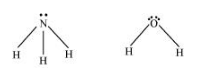# Although geometries of NH3 and H2O molecules are distorted tetrahedral,

Question:

Although geometries of $\mathrm{NH}_{3}$ and $\mathrm{H}_{2} \mathrm{O}$ molecules are distorted tetrahedral, bond angle in water is less than that of ammonia. Discuss.

Solution:

The molecular geometry of $\mathrm{NH}_{3}$ and $\mathrm{H}_{2} \mathrm{O}$ can be shown as:The central atom (N) in NHhas one lone pair and there are three bond pairs. In H2O, there are two lone pairs and two bond pairs.

The two lone pairs present in the oxygen atom of H2O molecule repels the two bond pairs. This repulsion is stronger than the repulsion between the lone pair and the three bond pairs on the nitrogen atom.

Since the repulsions on the bond pairs in H2O molecule are greater than that in NH3, the bond angle in water is less than that of ammonia.